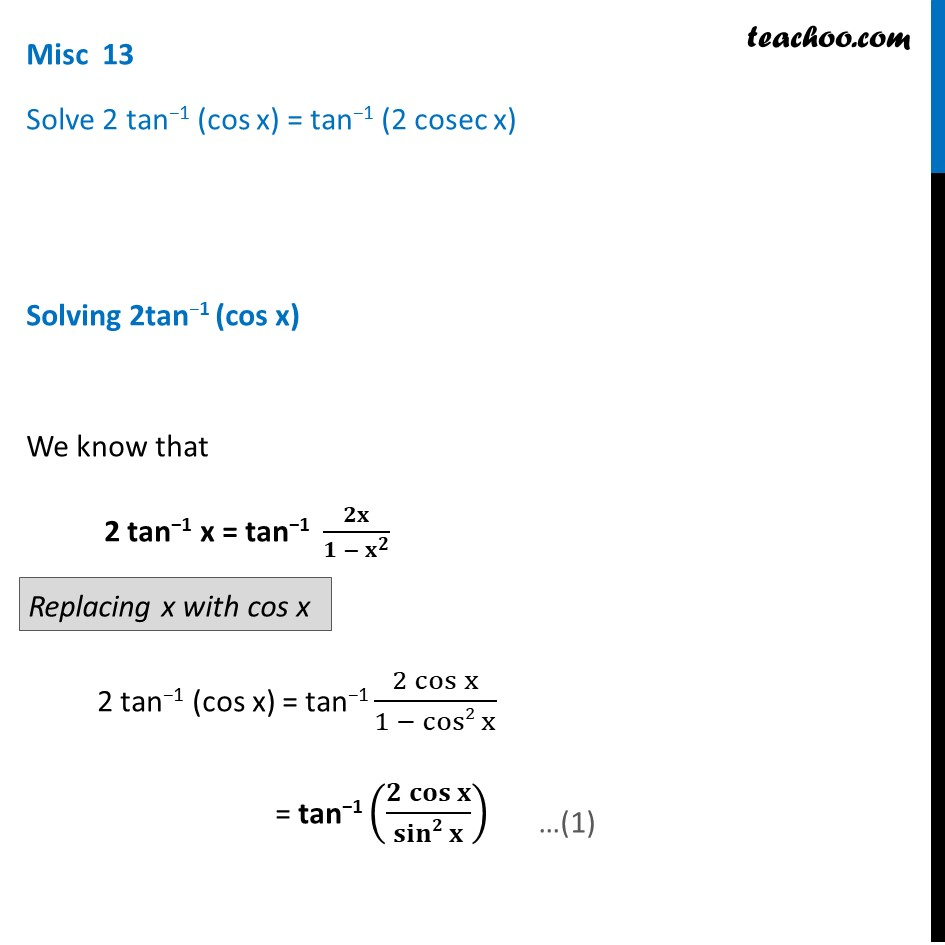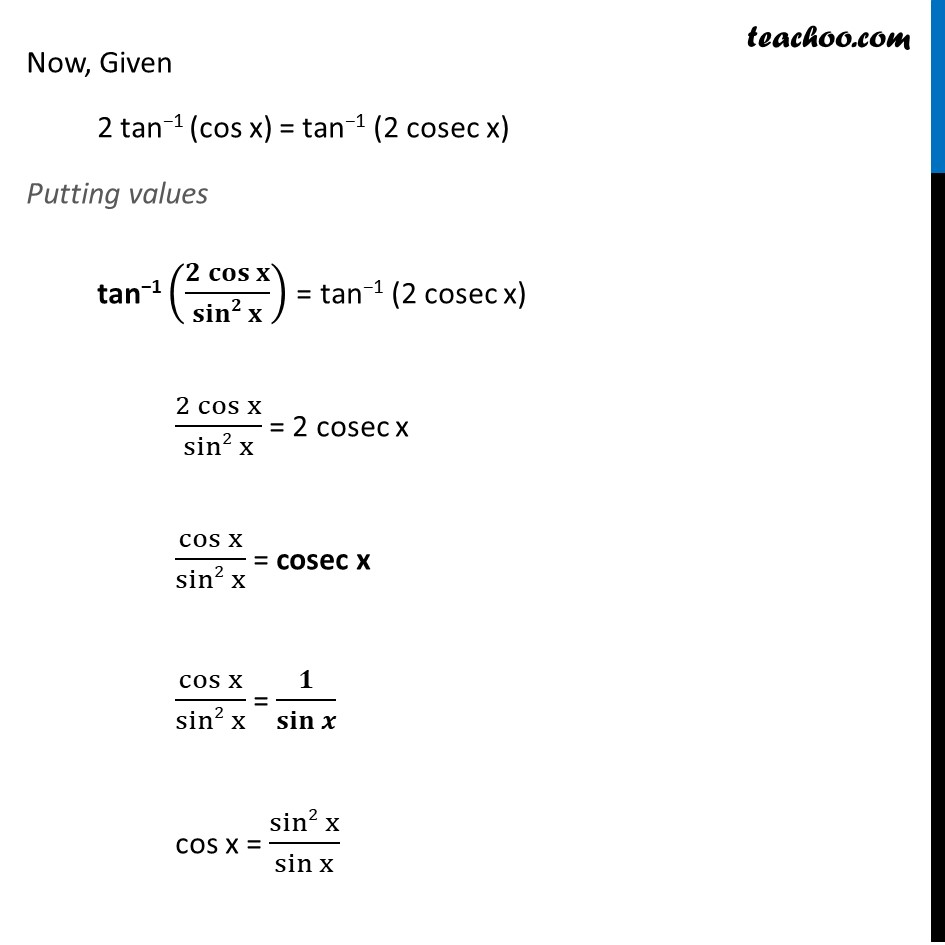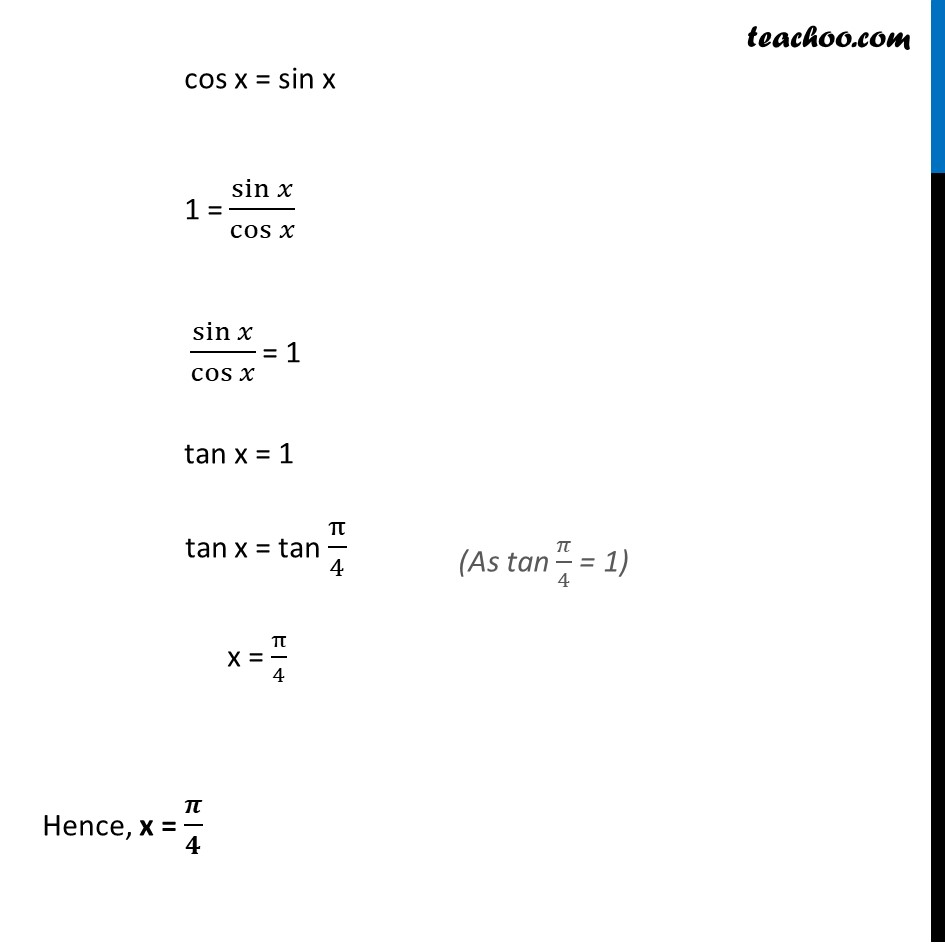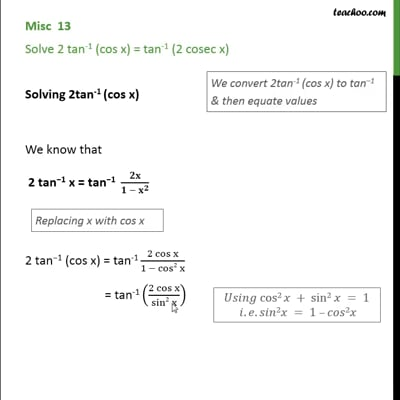Formulae based

Chapter 2 Class 12 Inverse Trigonometric Functions
Concept wiseThis video is only available for Teachoo black users

Solve all your doubts with Teachoo Black (new monthly pack available now!)

### Transcript

Misc 13 Solve 2 tan−1 (cos x) = tan−1 (2 cosec x) Solving 2tan−1 (cos x) We know that "2 tan−1 x = tan−1 " 𝟐𝐱/(𝟏 − 𝐱^𝟐 ) "2 tan−1 (cos x)" = tan−1 (2 cos⁡x)/(1 − cos2 x) = tan−1 ((𝟐 𝐜𝐨𝐬⁡𝐱)/(𝐬𝐢𝐧𝟐 𝐱)) Now, Given 2 tan−1 (cos x) = tan−1 (2 cosec x) Putting values tan−1 ((𝟐 𝐜𝐨𝐬⁡𝐱)/(𝐬𝐢𝐧𝟐 𝐱)) = tan−1 (2 cosec x) (2 cos⁡x)/(sin2 x) = 2 cosec x cos⁡x/(sin2 x) = cosec x cos⁡x/(sin2 x) = 𝟏/(𝐬𝐢𝐧 𝒙) cos x = (sin2 x)/(sin x) cos x = sin x 1 = sin⁡𝑥/cos⁡𝑥 sin⁡𝑥/cos⁡𝑥 = 1 tan x = 1 tan x = tan π/4 x = π/4 Hence, x = 𝝅/𝟒 (As tan 𝜋/4 = 1)